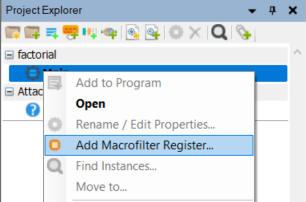You are here: Start » Tutorial Exercises » ADVANCED: Factorial (factorial)

## Aim

Create a macrofilter which computes a factorial for a given value.

The function argument should be provided as Integer.

## Input

Single integer for which factorial value should be computed.

## Output

Factorial value of the input value.

## Hints

This exercise should be solved using macrofilter's registers. All computed steps should be accumulated in a single macrofilter register.

Create the Task macrofilter which computes factorial value using the internal EnumerateIntegers filter.

## Solution (AVS)

1. Create a new Task macrofilter in PROCESS section and name it Factorial.2. Create an input inN of type Integer.

3. Enter to the Factorial macrofilter and add a new register regFactorial of type Long. Set its default value to 1 as on the image below.Configuring a macrofilter's register:4. Add filter EnumerateIntegers to Factorial macrofilter's ACQUIRE section and set inStart to 1.

5. Connect macrofilter's input inN with inCount.

6. Add a new Formula into the macrofilter's PROCESS section and connect outValue to it.

7. Connect prevFactorial to the formula.

8. Create a new output in the formula named outNextFactorial:

outNextFactorial = inValue * inPrevFactorial

9. Create a new macrofilter's output named outValue of type Long.

10. Connect the outNextFactorial formula's output with the macrofilter's outputs: nextFactorial and outValue.

11. Set a default output value of the outValue output to 1. This value will be set on the output when any iteration of the internal loop was performed.### Macrofilter Factorial computes a factorial value to using its registers.